# Change Nickname

Current Nickname:
Search
v4.0
v4.0

# PageRank & ArticleRank

## Overview

PageRank algorithm is an iterative algorithm that passes the scores of nodes along the direction of the directed edges in directed graph until the score distribution of the whole graph converges, the underlying assumption is that 'more important web pages are likely to receive more or more important backlinks from other web pages'. This algorithm was proposed from 1997 to 1998 by cofounders of Google, Larry Page and Sergey Brin, with the purpose to calculate the popularity and importance of web pages, so as to provide a basis for ranking search results. With the development of technology and the emergence of enormous correlation data, the usage of PageRank has been derived into many other fields.

ArticleRank is a variant of PageRank algorithm. Using Ultipa's PageRank algorithm, user may specify the calculation of PageRank or ArticleRank by modifying some parameter's configuration item.

Related materials of the algorithm:

## Basic Concept

The 1-step neighbor of a node that is connected with an outbound edge of that node is called the forward link of that node; on the contrary, it's called the backlink of that node if it's connected with an inbound edge of the node. When using node to represent web page, forward links of node are the web pages that are referenced by that web page, and backlinks represent the web pages that reference that web page.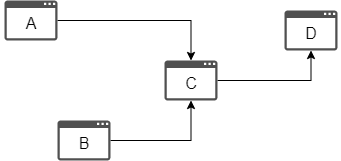A and B are backlinks of C, D is forward link of C

### PageRank

In the PageRank algorithm, all nodes are assigned an initial score; the score is evenly divided among all outbound edges of the node and passed to each forward link of that node; in the meantime, the node receives score from all its backlinks, and the sum of these received scores is the score the node gets in this round of transfer: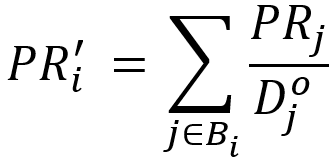In the formula above, node `j` is any backlink of node `i`, `D(j)` is the out degree of `j`.

### ArticleRank

The reference of publications is very similar to the reference of web pages, but there are also differences, for instance, a publication cannot reference itself, two publications cannot reference each other, and the references in a published publication do not change, etc. A publication reference graph has the following features: node does not have self-loop edge, a node cannot be both the forward link and the backlink of another node, and the forward links (out degree) of node will not change.

In comparison with PageRank, Ultipa's ArticleRank divides the score of node equally, not by the out degree (number of edges in the outbound direction) of that node, but "the sum of the out degree of that node and the average out degree of nodes in the whole graph" (which also differs from the original ArticleRank). Intuitively, this change will greatly weaken the score transfer capability of nodes with the out degree that is much lower than the average out degree of the whole graph.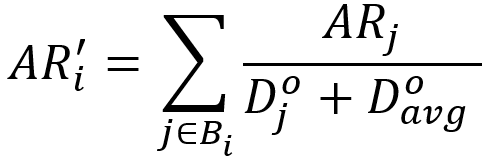In the formula above, `D(avg)` is the average out degree of the whole graph.

There is no circle in an ideal publication reference graph, thus the total score of the whole graph would decrease as the number of transfer rounds increases, and the score of ArticleRank will never become steady without a damping coefficient.

### Damping Coefficient

In PageRank algorithm, consider those web pages that are not referenced by any other sites (nodes with in degree of 0 and no backlink), such as those lonely web pages. Although the score they receive is always 0, they still need to be browsed in the real Internet. There are also some web pages do not reference any other sites (nodes with out degree of 0 and no forward link), the score transfer of the whole graph should not become meaningless due to the score loss on these nodes.

To deal with the two cases above as well as the problem that ArticleRank cannot converge, damping coefficient - a numeric value between 0 to 1 - is introduced to give each node a floor score while weakening the scores passed from the backlinks (if has) of the node. Take PageRank, for example, when the damping coefficient is `0.7`, each node gets a floor score of `1 - 0.7 = 0.3`, let's say the score a node receives is `8`, it will be weakened to `8 * 0.7 = 5.6`, and the sum of the two parts is the score of the node in this round: `0.3 + 5.6 = 5.9`.

Given `c` to represent the damping coefficient, the score of node after each round of transfer is: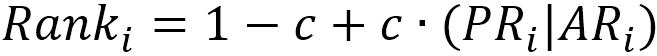Ultipa supports both PageRank and ArticleRank score calculation methods, which can be configured by algorithm parameters.

At the beginning of the algorithm, the initial score of all nodes is set according to the algorithm parameters; in each iteration, the score is calculated and updated for each node. Iterating and looping by this rule until the score of nodes in the whole graph no longer changes, or the number of iterations reaches the limit.

## Special Case

### Lonely Node, Disconnected Graph

With the damping coefficient `c`, the score lonely node gets is always `1-c` (except for the initial state).

There is no score transfer between different connected components in disconnected graph, the score transfer reaches steady state inside each connected component.

### Self-loop Edge

In PageRank algorithm, each self-loop edge is regarded as a valid out degree and a valid in degree, that is, self-loop edge of a node passes part of the score to the node itself, and the execution of this algorithm in a graph with self-loop edge usually requires more iterations to stabilize.

### Directed Edge

The score of nodes are divided and passed along the direction of directed edges in PageRank algorithm.

## Results and Statistics

Take the book reference graph below as an example, run the PageRank algorithm, the initial score of all nodes is 1, the damping coefficient is 0.8, use ArticleRank score calculation methods and to iterate 5 rounds: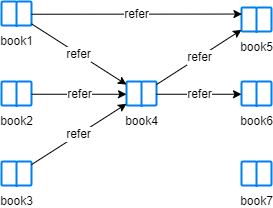Algorithm results: Calculate score for each node, return `_id`, `rank` or `_uuid`, `rank` according to the execution method

_uuid _id rank
7 book7 0.20000000
2 book2 0.20000000
1 book1 0.20000000
3 book3 0.20000000
4 book4 0.42830801
6 book6 0.31992599
5 book5 0.37592599

Algorithm statistics: N/A

## Command and Configuration

• Command: `algo(page_rank)`
• Configurations for the parameter `params()`:
Name
Type
Default
Specification
Description
init_value float 0.2 >0 Initial score
loop_num int 5 >=1 Number of iterations
damping float 0.8 0~1 Damping coefficient, i.e. the probability that users continue to stay on the current page
weaken int 1 1 or 2 1 or if not set means to calculate PageRank, 2 means to calculate ArticleRank
limit int -1 >=-1 Number of results to return; return all results if sets to -1 or not set
order string / ASC or DESC, case insensitive To sort the returned results; no sorting is applied if not set

Example: Run the PageRank algorithm, the initial score of all nodes is 1, the damping coefficient is 0.8, use ArticleRank score calculation methods and to iterate 5 rounds

``````algo(page_rank).params({
init_value: 1,
loop_num: 5,
damping: 0.8,
weaken: 2
}) as rank return rank
``````

## Algorithm Execution

#### 1. File Writeback

Configuration Data in Each Row
filename `_id`,`rank`

Example: Run the PageRank algorithm, the initial score of all nodes is 1, the damping coefficient is 0.8, use PageRank score calculation methods and to iterate 5 rounds, write the algorithm results back to CSV file named ranking

``````algo(page_rank).params({
init_value: 1,
loop_num: 5
}).write({
file:{
filename: "ranking.csv"
}
})
``````

#### 2. Property Writeback

Configuration Writeback Content Type Data Type
property `rank` Node property `float`

Example: Run the PageRank algorithm, the initial score of all nodes is 1, the damping coefficient is 0.8, use PageRank score calculation methods and to iterate 5 rounds, write the algorithm results back to node property named score

``````algo(page_rank).params({
init_value: 1,
loop_num: 5
}).write({
db:{
property: "score"
}
})
``````

#### 3. Statistics Writeback

This algorithm has no statistics.

### Direct Return

Alias Ordinal Type Description Column Name
0 []perNode Node and its score `_uuid`, `rank`

Example: Run the PageRank algorithm, the initial score of all nodes is 1, the damping coefficient is 0.8, use ArticleRank score calculation methods and to iterate 5 rounds, define algorithm results as alias named rank, and return the result

``````algo(page_rank).params({
init_value: 1,
loop_num: 5,
damping: 0.8,
weaken: 2
}) as rank return rank
``````

### Streaming Return

Alias Ordinal Type Description Column Name
0 []perNode Node and its score `_uuid`, `rank`

Example: Run the PageRank algorithm, the initial score of all nodes is 1, the damping coefficient is 0.8, use PageRank score calculation methods and to iterate 5 rounds, return the 3 results with the highest score

``````algo(page_rank).params({
init_value: 1,
loop_num: 5
}).stream() as score
order by score.rank desc
return score limit 3
``````

### Real-time Statistics

This algorithm has no statistics.